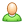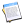Guest |Rus ||Selection RulesSelection Rulesversion 0.1

 Class Notation Process Restrictions (Selection Rules) (States) Selection Rules (Transitions) Selection Rules (MathML) Diatomic closed shell dcs Hund’s case (a) diatomics hunda Hund’s case (b) diatomics hundb Closed-shell, linear triatomic molecules ltcs ltcs_Notation_Dinf_h_Normal_Modes Absorption 1.  l2 = v2 2. J ≥ l2 3. if l2 =0,  then ε = e. (ε = ltcs:kronigParity) Permitted transitions ΔJ = 0 | -1 | +1 if ΔJ = 0,  then ltcs:kronigParity has to be changed  (e←f,   f←e) if ΔJ  =/= 0,  then  ltcs:kronigParity has not to be changed  (e←e   f← f) if  l2' = l2 " = 0, then independently at J - Q - branch is prohibited.  thansition permitted if  Δv2 +  Δv3 = 2n+1, n=0,1,2,3, .... ( Δvi = |vi' - vi"| ) Prohibited transitions      [ (-1)v3+v2 =1 и  ε(-1)J=-1]   или  [ (-1)v3+v2 =-1 и  ε(-1)J=1] here ε=1 in case of ltcs:kronigParity=e and ε=-1 in case of ltcs:kronigParity=f. ltcs_Notation_Cinf_v_Normal_Modes Absorption 1.  l2 = v2 2. J ≥ l2 3. if l2 =0,  then ε = e. (ε = ltcs:kronigParity) Permitted transitions ΔJ = 0 | -1 | +1 if ΔJ = 0,  then ltcs:kronigParity has to be changed  (e←f,   f←e) if ΔJ  =/= 0,  then  ltcs:kronigParity has not to be changed  (e←e   f←f) if  l2' = l2 " = 0, then independently at J - Q - branch is prohibited.  thansition permitted if  Δv2 +  Δv3 = 2n+1, n=0,1,2,3, .... ( Δvi = |vi' - vi"| ) ltcs_Notation_Dinf_h_CDSD Absorption Prohibited states (m(-1)J=-1 и (-1)P=1) or (m(-1)J=1 и (-1)P=-1), where m=1 for ltcs:kronigParity=e and m=-1 for  ltcs:kronigParity=f . Permitted transitions ΔJ = 0 | -1 | +1 if ΔJ = 0,  then ltcs:kronigParity has to be changed  (e  ←  f,   f ← e) if ΔJ  =/= 0,  then  ltcs:kronigParity has not to be changed  (e  ← e   f ← f) ΔP=P '' - P ' = 2n+1, n=0,1,2,... (P=ltcs_notation:P) ltcs_Notation_Cinf_v_CDSD Absorption Prohibited states (m(-1)J=-1 и (-1)P=1) or (m(-1)J=1 и (-1)P=-1), where m=1 for ltcs:kronigParity=e and m=-1 for  ltcs:kronigParity=f . Permitted transitions ΔJ = 0 | -1 | +1 if ΔJ = 0,  then ltcs:kronigParity has to be changed  (e  ←  f,   f ← e) if ΔJ  =/= 0,  then  ltcs:kronigParity has not to be changed  (e  ← e   f ← f) Closed-shell, non-linear triatomic molecules nltcs nltcs_Notation_C2v_Normal_Modes Absorption nltcs_Notation_Cs_Normal_Modes Absorption nltcs_Notation_C2v_BT2 Absorption nltcs_Notation_Cs_VTT Absorption Closed-shell, symmetric top molecules stcs Closed-shell, linear, polyatomic molecules lpcs Closed-shell, asymmetric top molecules asymcs Open-shell, asymmetric top molecules asymos Closed-shell, spherical top molecules sphcs Open-shell, spherical top molecules sphos Open-shell, linear triatomic molecules ltos Open-shell, linear, polyatomic molecules lpos Open-shell, non-linear triatomic molecules nltosГрант INTAS 00-189